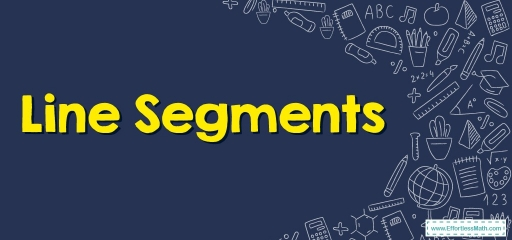# Line Segments

In this article, we want to introduce you to the line, line segment, ray, features of each, and their differences.Line segments are paths in-between $$2$$ points that you can measure. Because line segments have defined lengths, they can create the sides of polygons. It’s not the same as a line that doesn’t have any beginning or end and that can be expanded in $$2$$ directions.

Line segments are represented by a bar on top, that’s the symbol for line segments.

## Way to Measure a Line Segment?

A line segment can be measured with the assistance of a ruler (scale). Here’s the way to measure a particular line segment and call it $$PQ$$.

• Step one: Put the ruler’s tip carefully at the Zero so it is put at the beginning point $$P$$ of the particular line segment.
• Step two: Then, begin to read the values provided on the ruler and see which number comes on the additional endpoint $$Q$$.
• Step three: So, the line segment’s length equals four inches, which you can write as $$PQ¯$$ $$=$$ four inches.

## The formula for a Line Segment

In the example above, we calculated the line segment’s length as $$PQ$$ to be four inches. That is put down as $$PQ¯ = 4$$ inches. So, let’s find out the way to locate a line segment’s length whenever the coordinates of the $$2$$ endpoints are provided. In this instance, we utilize the distance formula, which is, D = $$\sqrt{(x2-x1)^2+ (y2-y1)^2}$$. Then, $$(x1, y1)$$ and $$(x2, y2)$$ are coordinates of the particular points.

### Line Segments – Example 1:

Determine whether the following shape is a line, a line segment, or a ray.

Solution:

This shape is a line segment because it is part of a line with a starting point and an endpoint.

### Line Segments – Example 2:

Determine whether the following shape is a line, a line segment, or a ray.

Solution:

This shape is a line because it is shown with arrows at both ends to signify it goes on forever.

## Exercises for Line Segments

Determine whether the following shapes are a line, a line segment, or a ray.

1)

2)

1. ray
2. line segment

### What people say about "Line Segments - Effortless Math: We Help Students Learn to LOVE Mathematics"?

No one replied yet.

X
30% OFF

Limited time only!

Save Over 30%

SAVE $5 It was$16.99 now it is \$11.99